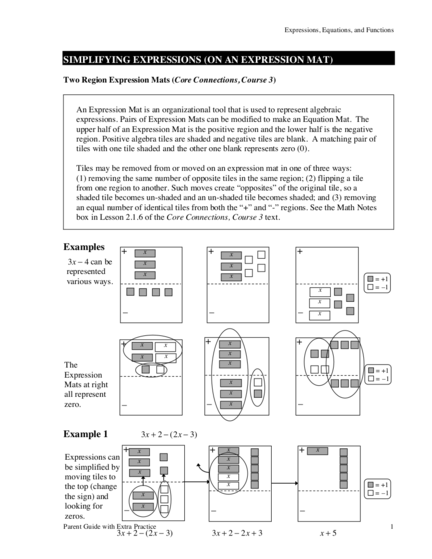# Learn Algebraic Expression SimplificationContributed by:Learn and practice simplifying algebraic expressions using an expression mat.
1. Expressions, Equations, and Functions
SIMPLIFYING EXPRESSIONS (ON AN EXPRESSION MAT)
Two Region Expression Mats (Core Connections, Course 3)
An Expression Mat is an organizational tool that is used to represent algebraic
expressions. Pairs of Expression Mats can be modified to make an Equation Mat. The
upper half of an Expression Mat is the positive region and the lower half is the negative
region. Positive algebra tiles are shaded and negative tiles are blank. A matching pair of
tiles with one tile shaded and the other one blank represents zero (0).
Tiles may be removed from or moved on an expression mat in one of three ways:
(1) removing the same number of opposite tiles in the same region; (2) flipping a tile
from one region to another. Such moves create “opposites” of the original tile, so a
an equal number of identical tiles from both the “+” and “-” regions. See the Math Notes
box in Lesson 2.1.6 of the Core Connections, Course 3 text.
Examples + x + +
x
can be x x
represented x x
various ways. = +1
x = –1
x
_ _ _ x
+ x x + x +
x x x
The x
= +1
Expression = –1
x
Mats at right
all represent x
zero. _ _ x _
Example 1 3x + 2 ! (2x ! 3)
+ x + x + x
Expressions can x x
be simplified by x
x
moving tiles to x
the top (change x = +1
x = –1
the sign) and
looking for _ x _ _
Parent Guide with Extra Practice 1
2. Example+ 2 1y! (2y ! 3) + y +
!2 y +
y
y y
= +1
y = –1
y
_ _ _
1 ! (2y ! 3) + y ! 2 1 ! 2y + 3 + y ! 2 !y + 2
Simplify each expression.
1. + 2. + 3. +
x
x
x
x y
y
_ _ _
4. 5. 6.
+ x x + +
y
x x
y
y
x
y
y
_ _ _
7. 3 + 5x ! 4 ! 7x 8. !x ! 4x ! 7 9. !(!x + 3)
10. 4x ! (x + 2) 11. 5x ! (!3x + 2) 12. x ! 5 ! (2 ! x)
13. 1 ! 2y ! 2y 14. !3x + 5 + 5x ! 1 15. 3 ! (y + 5)
16. !(x + y) + 4x + 2y 17. 3x ! 7 ! (3x ! 7) 18. !(x + 2y + 3) ! 3x + y
2 Core Connections, Courses 1–3
3. Expressions, Equations, and Functions
1. 0 2. 2x + 2 3. 2y + 2
4. !5x + 2 5. 2y ! 1 6. !y + 5
7. !2x ! 1 8. !5x ! 7 9. x!3
10. 3x ! 2 11. 8x ! 2 12. 2x ! 7
13. !4y + 1 14. 2x + 4 15. !y ! 2
16. 3x + y 17. 0 18. !4x ! y ! 3
Parent Guide with Extra Practice 3